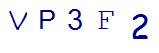Last Updated on August 31, 2021 by Thinkster

Fourth grade brings many significant changes to math class. Problems get harder and your student starts bringing home more and more worksheets in this grade. If you have a child heading into this challenging grade, what type of problems can you expect to see? Here are some of the most important 4th grade math worksheets, and some explanation as to why these concepts are so important for your child to master.

### Multiplication and Division Worksheets

Multiplication and division are a common part of 4th grade math worksheets. These worksheets become increasingly complex, with the introduction of multiplication of multi-digit numbers and long division problems with remainders or decimals. Your fourth grader will also need to be able to understand what multiplication and division means. When the student sees 5 times 7 equals 35, he should be able to state that this means 35 is 5 times as many as 7, and vice versa.

### Word Problem Worksheets

Fourth grade is the year that teachers try to show students how math is seen in everyday life. Because of this, many 4th grade math worksheets present real-world problems, especially word problems, that put math concepts into real-world situations. Sometimes students will have trouble applying math to word problems, and this is an area where your fourth grader may need a little extra help.

### Fraction Worksheets

Many 4th grade math worksheets involve the fraction problems. Fourth graders start comparing, adding, subtracting, multiplying, dividing and reducing fractions. They will also start changing fractions into decimals. All of these operations rely on a good, solid foundation in basic math processes. Someone who struggles with division will struggle even more with converting fractions into decimals.

### Geometry Worksheets

Geometry is also explored more in-depth in the fourth grade. Expect to see your child bring home worksheets with angles to measure, shapes to identify, including 3D shapes, types of triangles and finding the area and perimeter of a variety of shapes. These skills provide a foundation for geometry class in high school and are critical skills to master.

If you find that a certain type of worksheet is making your child struggle, there are ways you can help. Instead of throwing more 4th grade math worksheets to your child, find an iPad-based tutoring program. A tablet-based learning app will turn the math work into something fun and inviting for your student to tackle. With the help of a program designed by teachers, you can give your child the practice he or she needs to succeed in 4th grade math.

SummaryArticle Name
Description
Here are some of the most important 4th grade math worksheets, and some explanation as to why these concepts are so important for your child to master.
Author
Publisher Name
Thinkster Math
Publisher Logo

## Subscribe to Thinkster Blog## Recommended Articles### Unlocking the Code: Teaching Mathematical Symbols and Their Meaning

Knowledge of mathematical symbols is a foundational skill that empowers students to engage with and excel i...